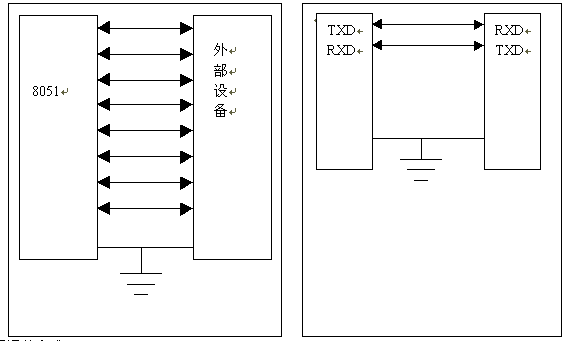# 8051单片机(STC89C52)两个倒计时器同步计数

2020-08-02来源: eefocus

QQ#include

void delay()//用定时器0实现5ms精准定时

{

TMOD = 0x01;

TH0 = 0xEA;

TL0 = 0x84;//65536 - 5000 = 60036

TF0 = 0;//置溢出标志位为0

TR0 = 1;//开启定时器0

while(TF0 == 0);

TR0 = 0;//暂停定时器0

}

void disp_digit(int d, int r)

{

unsigned char code DIG_CODE = {0x3f, 0x06, 0x5b, 0x4f, 0x66, 0x6d, 0x7d, 0x07, 0x7f, 0x6f};

//第一个倒计时

//显示个位数

P2 = 0;// P2 = 0 -> (P24, P23, P22) = (0, 0, 0) -> 右数第一个数字点亮

P0 = DIG_CODE[d % 10];

delay();//5ms精准定时

//显示十位数

P2 = 1 << 2;//P2 = 0000 0100 -> (P24, P23, P22) = (0, 0, 1) -> 右数第二个数字点亮

P0 = DIG_CODE[d / 10];

delay();//5ms精准定时

//第二个倒计时

//显示个位数

P2 = 6 << 2;//P2 = 0001 1000 -> (P24, P23, P22) = (1, 1, 0) -> 右数第七个数字点亮

P0 = DIG_CODE[r % 10];

delay();//5ms精准定时

//显示十位数

P2 = 7 << 2;//P2 = 0001 1100 -> (P24, P23, P22) = (1, 1, 1) -> 右数第八个数字点亮

P0 = DIG_CODE[r / 10];

delay();//5ms精准定时

}

int main()

{

int i;

int sec1, sec2;

while(1)

{

sec1 = 15;

sec2 = 15;

while(sec1 >= 0)

{

for(i = 0; i < 50; i++)

{

disp_digit(sec1, sec2);//花费大约20ms

}//20ms*50=1000ms=1s

sec1 --;

sec2 --;

}

}

return 0;

}北京航空航天大学教授，20余年来致力于单片机与嵌入式系统推广工作。北京市海淀区知春路23号集成电路设计园量子银座1305 电话:(010)82350740 邮编：100191